+

# Bar Graphs and Pie Charts

Author: Sophia Tutorial
##### Description:

Calculate the percentage of categorical or qualitative data for a pie chart.

(more)

Sophia’s self-paced online courses are a great way to save time and money as you earn credits eligible for transfer to many different colleges and universities.*

No credit card required

37 Sophia partners guarantee credit transfer.

299 Institutions have accepted or given pre-approval for credit transfer.

* The American Council on Education's College Credit Recommendation Service (ACE Credit®) has evaluated and recommended college credit for 32 of Sophia’s online courses. Many different colleges and universities consider ACE CREDIT recommendations in determining the applicability to their course and degree programs.

Tutorial
what's covered
This tutorial will cover how to display qualitative data utilizing bar graphs and pie charts. We will explore how to create and interpret bar graphs and pie charts, discussing the following:

1. Bar Graphs
2. Bar Graphs: Relative Frequency
3. Multiple Bar Graphs
4. Pie Charts

## 1. Bar Graphs

Categorical data is qualitative data, and it can be displayed visually in a bar graph, which will compare the number of values in each category. A bar graph is not the only way to display categorical data, but it is a common way.

Suppose there are 2070 students enrolled in the following college courses, which are taken by different majors. At this particular college, there are 331 economics majors, 435 biology majors, 124 chemistry majors, etc.

Course Frequency
Economics 331
Biology 435
Chemistry 124
Statistics 248
Psychology 311
Sociology 248
Spanish 207
History 166

You begin by drawing a horizontal axis and labeling the categories beneath it. You could also label it on the vertical axis and label the categories from top to bottom if you want. So, you wrote economics, biology, chemistry, and visually separated them.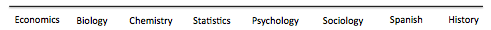Then, you need to create a vertical axis with frequency on it. The highest number in the data set is 435, so a smart choice would be to have the vertical axis frequencies go up to 450, or even 500. This way, all values will be represented.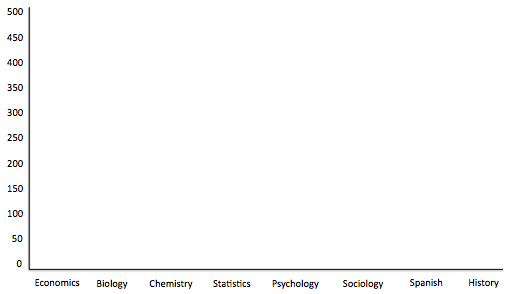Finally, you set up a bar that goes up to the number that corresponds to that category. Therefore, economics will have a bar that goes up to 331, biology will go all the way up to almost 450, etc.

The full bar graph looks like this: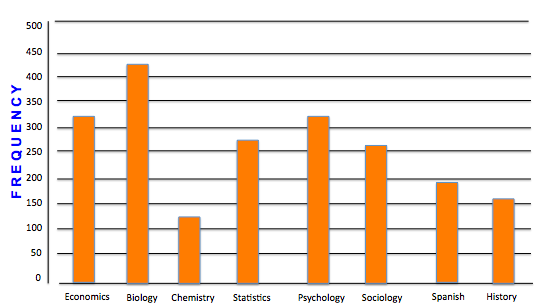term to know
Bar Graph
A distribution of qualitative data that displays bars that are proportional in length to the frequency or relative frequency of a particular data value

## 2. Bar Graphs: Relative Frequency

You can also use a bar graph to show relative frequency. Relative frequency shows how much of the whole each class represents. It is the percent of the values that are in each category.

How do you calculate relative frequency? Take each number, and divide it by the total number.

Course Frequency Relative Freq
Economics 331 /2070 = 16%
Biology 435 /2070 = 21%
Chemistry 124 /2070 = 6%
Statistics 248 /2070 = 12%
Psychology 311 /2070 = 15%
Sociology 248 /2070 = 12%
Spanish 207 /2070 = 10%
History 166 /2070 = 8%

There are 435 out of 2070 students in Biology, which means that Biology students make up 435/2070 = 21% of the total students. So you can see that the biology bar has just over 20% of the students.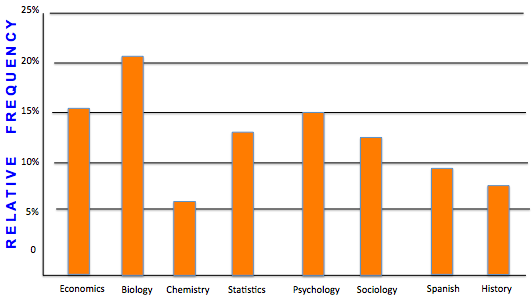Notice that in the previous example with counts, and this example with relative frequency, the shape and size of the bars didn't change. The only thing that changed was the vertical axis and what it was measuring.

## 3. Multiple Bar Graphs

Suppose that you wanted to know about the work habits of college students so you sample of 100 students. Perhaps you want to know if they were male or female, and whether they did not work at all, worked during summer only, or had a job all year long.

The following table shows the data collected in this sample.

Male Female
No Job 25 28
Summer Only 17 10
Job All Year 11 9

You can create multiple bar graphs on the same set of axes and compare them by category. One way to display these items in a bar graph would be to break it up by male and female. You choose green to be males and yellow to be females.

Next, you would break the horizontal axis into no job, summer only, and job all year.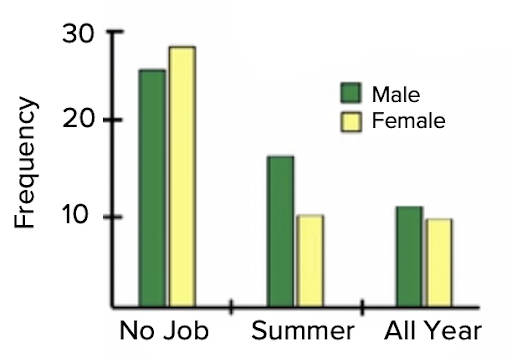Both bar graphs would be presented side-by-side within each category. There are 25 males who had no job and 29 females who had no job, etc.

What this one tells us is that males are more likely than females to have a job all year and in summer, and a little bit less likely than females to never have had a job.

The other way would be to flip-flop which category is represented by the colors and which category goes on the axis. Male and female could go on the axis, and the job status could be the colors. In that case, it would look like the following graph: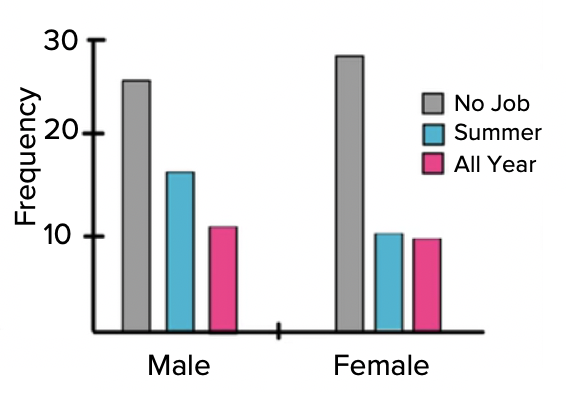This graph will tell you that both males and females have a tendency towards not having a job, then have a summer job or have a job all year.

## 3. Pie Charts

As mentioned, qualitative data can be displayed in a couple of different ways. As discussed above, one way is to display it in a bar graph. Another way to display it is with a pie chart. A pie chart displays relative frequencies for each category, which considers how these categories relate to the whole.

Let's use the same set of information from the first example with the 2070 students enrolled in college courses.

To make a pie chart, the first thing to do is to calculate relative frequencies. Remember, relative frequency is the percent of the values that are in each category and can be calculated by dividing each data value by the total number.

Course Frequency Relative Freq
Economics 331 /2070 = 16%
Biology 435 /2070 = 21%
Chemistry 124 /2070 = 6%
Statistics 248 /2070 = 12%
Psychology 311 /2070 = 15%
Sociology 248 /2070 = 12%
Spanish 207 /2070 = 10%
History 166 /2070 = 8%

Now its time to create the pie chart by following the steps below:

Step 1: First, find the relative frequency.

Step 2: Next, calculate the central angle for each category using the relative frequency. You may recall there are 360 degrees in a circle. The central angle for economics has to be 16 percent of the circle. So how do you set this up? You need 16% of 360 degrees. Multiply each percent by 360.

hint
Remember, when you multiply this out, it needs to be 0.16 because it's 16%.

Course Frequency Relative Freq Angle
Economics 331 ÷ 2070 = 16% x 360 = 57.6°
Biology 435 ÷ 2070 = 21% x 360 = 75.6°
Chemistry 124 ÷ 2070 = 6% x 360 = 21.6°
Statistics 248 ÷ 2070 = 12% x 360 = 43.2°
Psychology 311 ÷ 2070 = 15% x 360 = 54°
Sociology 248 ÷ 2070 = 12% x 360 = 43.2°
Spanish 207 ÷ 2070 = 10% x 360 = 36°
History 166 ÷ 2070 = 8% x 360 = 28.8°

0.16 times 360 gives you about 58 degrees. Therefore, your central angle, representing economics, with be approximately 58 degrees. Do the same thing with the remainder of the categories to obtain angle measurements for each of these central angles.

Step 3: Once you have determined the relative frequency of each category, you can create the sectors of your pie chart, shown below: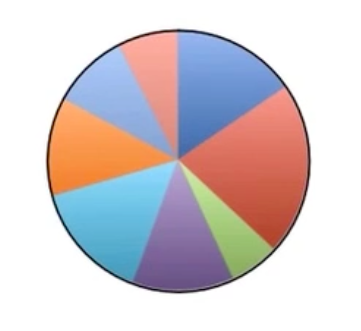But which sector corresponds to which category? You could write the words inside each sector, labeling each with the names of the majors. It's fairly clear that the biggest slice is biology. But which ones are the rest? You need to create a key.

Step 4: Finally, add a key off to the side. You can either have written the word economics within the blue sector, or you can create a blue square and write "Economics" next to it. That shows that anything that's blue means economics. You can do the same for each of the sectors.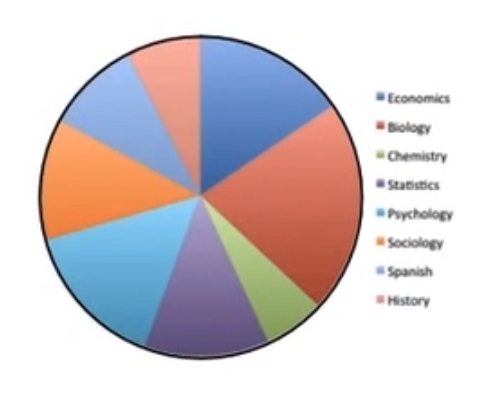step by step
Step 1: Find relative frequency
Step 2: Calculate the central angle using the relative frequency.
Step 3: Create the sectors
term to know
Pie Chart/Circle Graph
A distribution of qualitative data that shows the relative frequency of each category as a sector of a circle.

summary
Bar graphs are a nice way to view categorical data or the counts in a category for a qualitative data set. We can use frequencies or relative frequencies, if there's no overlap between the categories, to show how each category relates to the others. We can also put multiple bar graphs on the same set of axes. Pie charts are another form of visual display used for qualitative data. They display the relative frequency or percent of each category by dividing a circle into sectors that relate those relative sizes. The biggest advantage of a pie chart over a bar graph is that it is possible to see how each category relates to the whole. It's important to note that sometimes, because of rounding, the relative frequencies don't add up to 100%. They might add up to only 99% or 101%, as they did in our example. However, as long as the relative sizes of each are in the right proportions, it is not a major cause for concern. You can also fix this issue by rounding your values more precisely.

Good luck!

Source: Adapted from Sophia tutorial by Jonathan Osters.

Terms to Know
Bar Graph

A distribution of qualitative data that displays bars that are proportional in length to the frequency or relative frequency of a particular data value

Pie Chart/Circle Graph

A distribution of qualitative data that shows the relative frequency of each category as a sector of a circle.

Rating